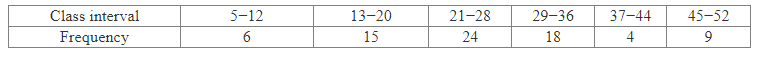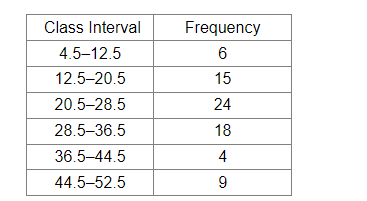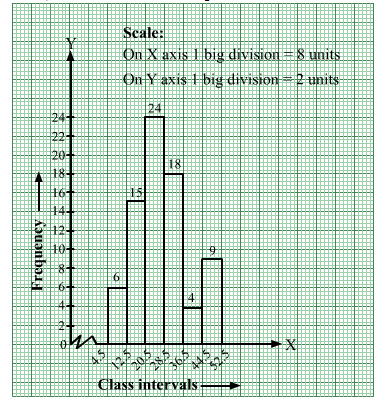# Construct a histogram for the following frequency distribution.

Question:

Construct a histogram for the following frequency distribution.Solution:

The given frequency distribution is in inclusive form.
So, we will convert it into exclusive form, as shown below:We will mark class intervals along the x-axis and frequencies along the y-axis.
The scale is as follows:
On x-axis: 1 big division = 8 units
On y-axis: 1 big division = 2 units
Because the scale on the x-axis starts at 4.5, a kink, i.e., a break, is indicated near the origin to signify that the graph is drawn with a scale beginning at 4.5
and not at the origin.
We will construct rectangles with class intervals as bases and the corresponding frequencies as heights.
Thus, we will obtain the histogram as shown below: